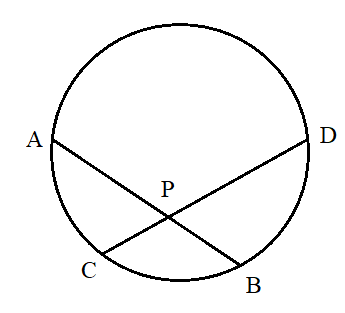Filters
Latest Questions
Mathematics
Chord of a circle
A chord of a circle of radius 10 cm subtends a right angle at its center. The length of chord (in cm) is
$a.{\text{ 5}}\sqrt 2 \\ b.{\text{ 10}}\sqrt 2 \\ c.{\text{ }}\dfrac{5}{{\sqrt 2 }} \\ d.{\text{ 10}}\sqrt 3 \\$
Mathematics
Chord of a circle
How do you find the length of the chord of a circle with radius $8\,cm$ and a central angle of $110^\circ$.
Mathematics
Chord of a circle
The sum of the squares of the length of the chords intercepted on the circle, ${{x}^{2}}+{{y}^{2}}=16$ , by the lines $x+y=n,\text{ }n\in N$ where $N$ is the set of all natural number , is:
(a) 320
(b) 160
(c) 105
(d) 210
Mathematics
Chord of a circle
If two distinct chords, drawn from the point $\left( {p,q} \right)$ on the circle ${x^2} + {y^2} = px + qy$. Given that: $pq \ne 0$ are bisected by the x-axis, then
A. ${p^2} < 8{q^2}$
B. ${p^2} > 8{q^2}$
C. Cannot be determined
D. None of these
Mathematics
Chord of a circle
If radii of two concentric circles are $4\text{ }cm$ and $\text{5 }cm$ then the length of each chord of one circle which is tangent to the other circle is:
A). $\text{3 }cm$
B). $\text{6 }cm$
C). $\text{9 }cm$
D). $\text{1 }cm$

Mathematics
Chord of a circle
Find the length of the longest chord of the circle in cm, if the radius of the circle is 2.9 cm.
Mathematics
Chord of a circle
If two distinct chord, drawn from the point (p, q) on the circle ${{x}^{2}}+{{y}^{2}}=px+qy$, where $pq\ne 0$ are bisected by the x – axis, then
(a) ${{p}^{2}}={{q}^{2}}$
(b) ${{p}^{2}}=8{{q}^{2}}$
(c) ${{p}^{2}}<8{{q}^{2}}$
(d) ${{p}^{2}}>8{{q}^{2}}$

Mathematics
Chord of a circle
Two chords AB and CD of lengths 5 cm and 11 cm respectively of a circle are parallel to each other and are on opposite sides of its center. If the distance between AB and CD is 6 cm, then find the radius of the circle?

Mathematics
Chord of a circle
Two congruent circles of radius r intersect such that each passes through the center of the other, then the length of the common chord is given by?
A. $r$
B. $2r$
C. $\sqrt 2 r$
D. $\sqrt 3 r$
Mathematics
Chord of a circle
If O is the centre of a circle, AB its chord, C is the midpoint of AB, then OAC is
A. An acute angle
B. An obtuse angle
C. A right angled triangle
D. An isosceles triangle
Mathematics
Chord of a circle
Chords AB and CD of a circle meet inside the circle at P. If PA $= 4cm$ , AB $= 7cm$ and PD $= 6cm$ , then length of CD isMathematics
Chord of a circle
What is the length of the common chord of two circles of radii 15 cm and 20 cm whose centres are 25 cm apart?
A. 24
B. 15
C. 12
D. none of the above
Prev
1
2
3
4
5